Anzeige

# Quiz manual (simple calculated question)

15. Oct 2012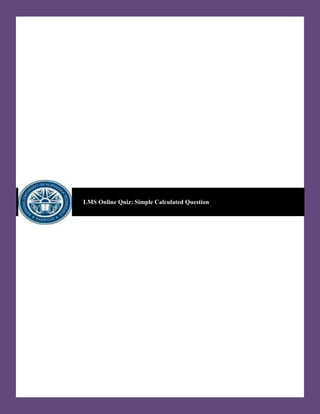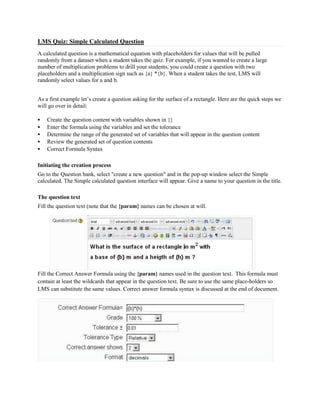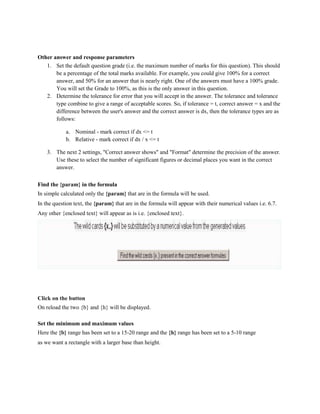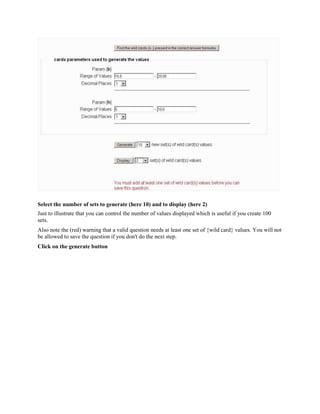Anzeige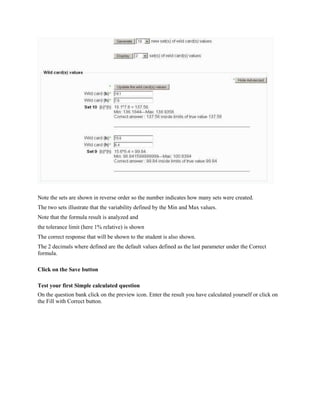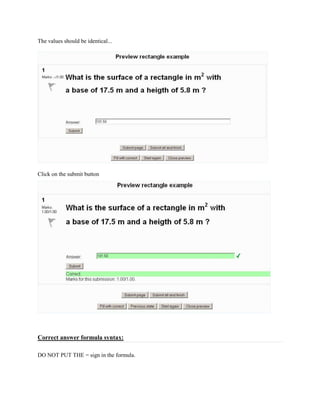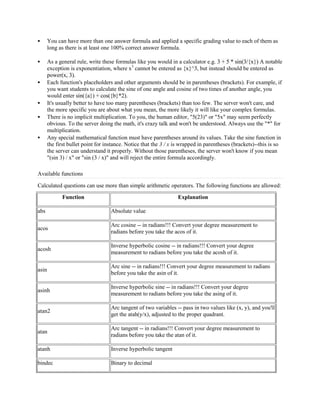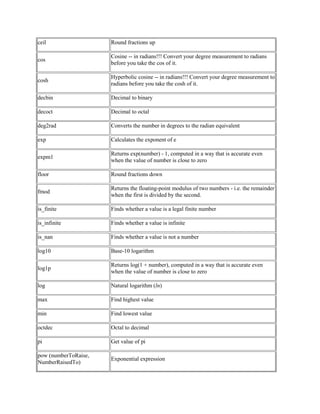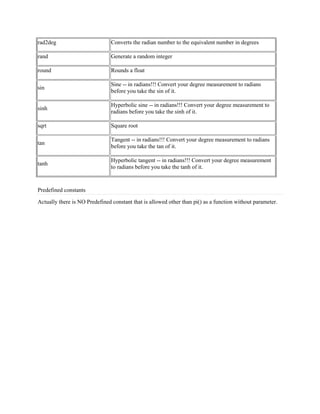Nächste SlideShareTeorema balzano weierstrass
1 von 9
Anzeige

### Quiz manual (simple calculated question)

1. LMS Online Quiz: Simple Calculated Question
2. LMS Quiz: Simple Calculated Question A calculated question is a mathematical equation with placeholders for values that will be pulled randomly from a dataset when a student takes the quiz. For example, if you wanted to create a large number of multiplication problems to drill your students, you could create a question with two placeholders and a multiplication sign such as {a} *{b}. When a student takes the test, LMS will randomly select values for a and b. As a first example let’s create a question asking for the surface of a rectangle. Here are the quick steps we will go over in detail:  Create the question content with variables shown in {}  Enter the formula using the variables and set the tolerance  Determine the range of the generated set of variables that will appear in the question content  Review the generated set of question contents  Correct Formula Syntax Initiating the creation process Go to the Question bank, select "create a new question" and in the pop-up window select the Simple calculated. The Simple calculated question interface will appear. Give a name to your question in the title. The question text Fill the question text (note that the {param} names can be chosen at will. Fill the Correct Answer Formula using the {param} names used in the question text. This formula must contain at least the wildcards that appear in the question text. Be sure to use the same place-holders so LMS can substitute the same values. Correct answer formula syntax is discussed at the end of document.